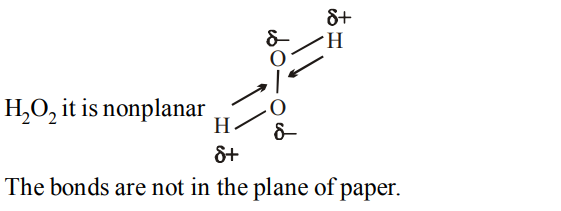## Chemical Bonding and Molecular Structure Questions and Answers Part-7

1. In which of the following pairs hydrogen-bonding is not possible ?
a) $NH_{3},NH_{3}$
b) $NH_{3},CH_{4}$
c) $H_{2}O,CH_{3}OCH_{3}$
d) $CH_{3}OH,CH_{3}OCH_{3}$

Explanation: In H - bonding atoms involved are F, O and N

2. The high density of water compared to ice is due to
a) H - bonding interactions
b) dipole - dipole interactions
c) dipole-induced dipole interactions
d) induced dipole-induced dipole interactions

Explanation: H -bonding interactions

3. Two ice cubes are pressed over each other until they unite to form one block. Which one of the following forces dominate for holding them together?
a) Dipole-dipole interaction
b) Van der waals’ forces
c) Hydrogen bond formation
d) Covalent attraction

Explanation: It is due to H - bonding

4. Which of the following molecules will form a linear polymeric structure due to hydrogen bonding ?
a) HCI
b) HF
c) $H_{2}O$
d) $NH_{3}$

Explanation: In HCl No–H–bonding, H – F form zig zag; (cage like by H2O, only NH3 forms linear polymeric structures)

5. Among $H_{2}O$ , $H_{2}S$ , $H_{2}Se$  and $H_{2}Te$ , the one with the highest boiling point is
a) $H_{2}O$  because of hydrogen bonding
b) $H_{2}Te$  because of higher molecular weight
c) $H_{2}S$  because of hydrogen bonding
d) $H_{2}Se$  because of lower molecular weight

Explanation: H2O

6. Which of the following is soluble in water ?
a) $CS_{2}$
b) $C_{2}H_{5}OH$
c) $CCI_{4}$
d) $CHCI_{3}$

Explanation: $C_{2}H_{5}OH$   soluble in water due to H-Bonding

7. A metallic bond is
a) ionic
b) polar covalent
c) non-polar covalent
d) electrostatic in nature

Explanation: Metallic bond is electrostatic in nature

8. Type of forces between molecules of $C_{6}H_{6}$ are
a) dipole-dipole interaction
b) dispersive forces
c) H-bonding
d) van der Waals’ forces

Explanation: Non polar molecules have dispersive forces

9. Which of the following has the highest dipole moment?
a) $AsH_{3}$
b) $SbH_{3}$
c) $PH_{3}$
d) $NH_{3}$

a) $H_{2}O_{2}$
b) $CH_{4}$
c) $C_{2}H_{6}$
d) $BF_4^-$